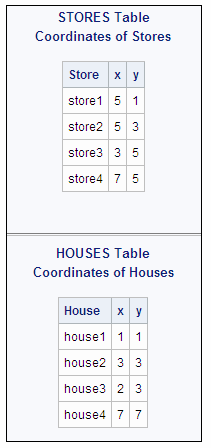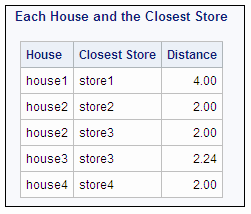# Example 12: Joining Two Tables and Calculating a New Value

 Features: GROUP BY clause HAVING clause SELECT clause : ABS function FORMAT= column-modifier LABEL= column-modifier MIN summary function ** operator, exponentiation SQRT function Table names: STORESHOUSES

### Details

This example joins two tables in order to compare and analyze values that are unique to each table yet have a relationship with a column that is common to both tables.
```proc sql;
title  'STORES Table';
title2 'Coordinates of Stores';
select * from stores;
title  'HOUSES Table';
title2 'Coordinates of Houses';
select * from houses;
title;```
The tables contain X and Y coordinates that represent the location of the stores and houses.
STORES and HOUSES Tables## Program

```proc sql;
title 'Each House and the Closest Store';
select house, store label='Closest Store',
sqrt((abs(s.x-h.x)**2)+(abs(h.y-s.y)**2)) as dist
label='Distance' format=4.2
from stores s, houses h```
```group by house
having dist=min(dist);```

## Program Description

Specify the query.The SELECT clause specifies three columns: HOUSE, STORE, and DIST. The arithmetic expression uses the square root function (SQRT) to create the values of DIST, which contain the distance from HOUSE to STORE for each row. The double asterisk (**) represents exponentiation. LABEL= assigns a label to STORE and to DIST.
```proc sql;
title 'Each House and the Closest Store';
select house, store label='Closest Store',
sqrt((abs(s.x-h.x)**2)+(abs(h.y-s.y)**2)) as dist
label='Distance' format=4.2
from stores s, houses h```
Organize the data into groups and subset the query.The minimum distance from each house to all the stores is calculated because the data are grouped by house. The HAVING clause specifies that each row be evaluated to determine whether its value of DIST is the same as the minimum distance from that house to any store.
```group by house
having dist=min(dist);```

## Output

Note that two stores are tied for shortest distance from house2.
New Value, Distance, Calculated from Two Tables